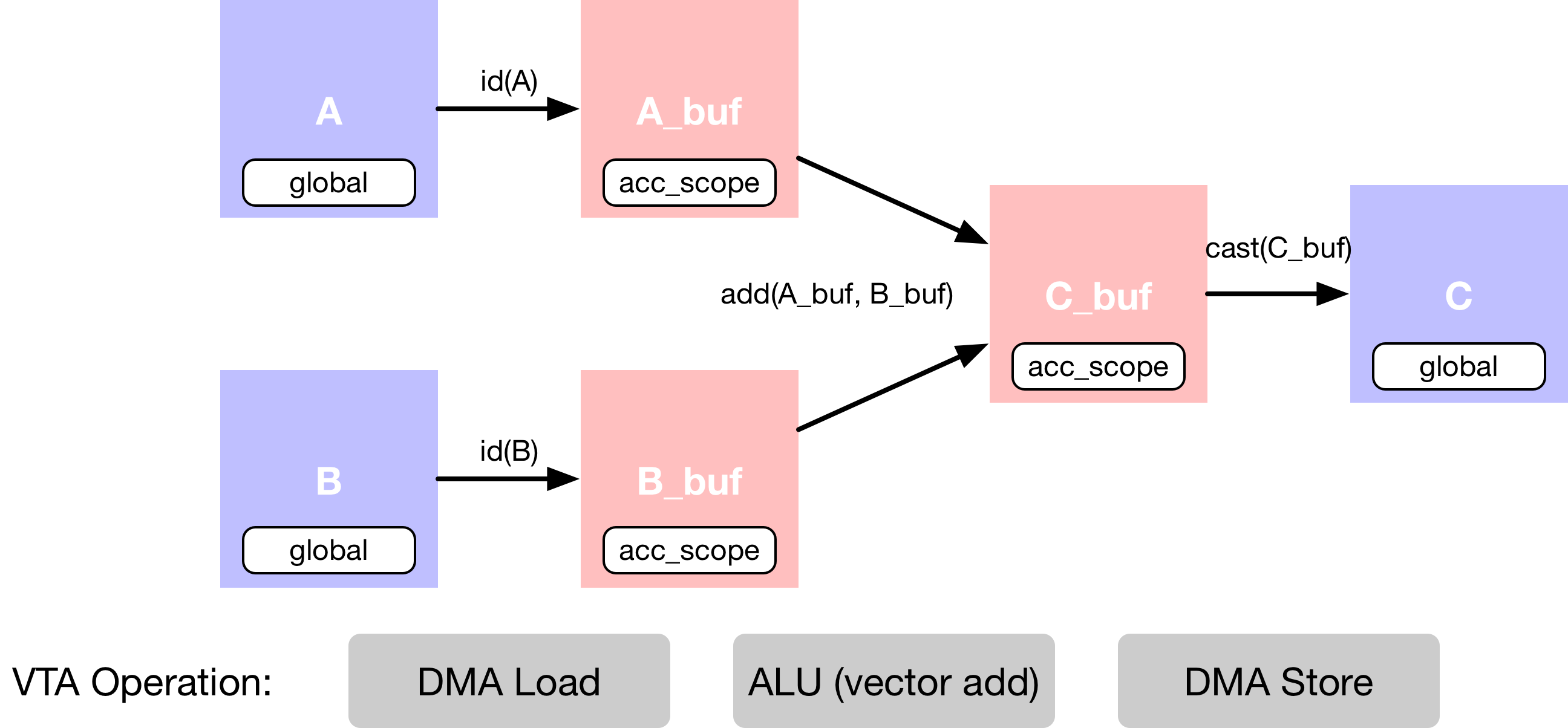# VTA 入门

``from __future__ import absolute_import, print_functionimport osimport tvmfrom tvm import teimport vtaimport numpy as np``

## 加载 VTA 参数​

VTA 的设计遵循模块化和可定制的原则。因此，用户可以自由修改影响硬件设计布局的高级硬件参数。这些参数在 `vta_config.json` 文件中由它们的 `log2` 值指定。这些 VTA 参数可以通过 `vta.get_env` 函数加载。

``env = vta.get_env()``

### FPGA 编程​

``# 要 TVM RPC 模块和 VTA 模拟器模块from tvm import rpcfrom tvm.contrib import utilsfrom vta.testing import simulator# 从 OS 环境中读取 Pynq RPC 主机 IP 地址和端口号host = os.environ.get("VTA_RPC_HOST", "192.168.2.99")port = int(os.environ.get("VTA_RPC_PORT", "9091"))# 在 Pynq 上配置比特流和 runtime 系统# 匹配 vta_config.json 文件指定的 VTA 配置。if env.TARGET == "pynq" or env.TARGET == "de10nano":    # 确保 TVM 是使用 RPC=1 编译的    assert tvm.runtime.enabled("rpc")    remote = rpc.connect(host, port)    # 重新配置 JIT runtime    vta.reconfig_runtime(remote)    # 使用预编译的 VTA 比特流对 FPGA 进行编程。    # 可以通过传递比特流文件的路径而非 None，    # 使用自定义比特流对 FPGA 进行编程    vta.program_fpga(remote, bitstream=None)# 在模拟模式下，本地托管 RPC 服务器。elif env.TARGET in ("sim", "tsim", "intelfocl"):    remote = rpc.LocalSession()    if env.TARGET in ["intelfocl"]:        # 对 intelfocl aocx 编程        vta.program_fpga(remote, bitstream="vta.bitstream")``

### 计算声明​## 输入占位符​

``# 输出通道因子 m - 总共 64 x 16 = 1024 输出通道m = 64# Batch 因子 o - 总共 1 x 1 = 1o = 1# A 平铺数据格式的占位符张量A = te.placeholder((o, m, env.BATCH, env.BLOCK_OUT), name="A", dtype=env.acc_dtype)# B 平铺数据格式的占位符张量B = te.placeholder((o, m, env.BATCH, env.BLOCK_OUT), name="B", dtype=env.acc_dtype)``

## 拷贝缓冲区​

``# A 复制缓冲区A_buf = te.compute((o, m, env.BATCH, env.BLOCK_OUT), lambda *i: A(*i), "A_buf")# B 复制缓冲区B_buf = te.compute((o, m, env.BATCH, env.BLOCK_OUT), lambda *i: B(*i), "B_buf")``

## 向量加法​

``# 描述 in-VTA 向量加法C_buf = te.compute(    (o, m, env.BATCH, env.BLOCK_OUT),    lambda *i: A_buf(*i).astype(env.acc_dtype) + B_buf(*i).astype(env.acc_dtype),    name="C_buf",)``

## 转换结果​

VTA 的特点之一是它只支持窄的 `env.inp_dtype` 数据类型格式的 DRAM 存储。这减少了内存传输的数据占用时间（在基本矩阵乘法示例中，对此进行了更多说明）。

``# 转换为输出类型，并发送到主存C = te.compute(    (o, m, env.BATCH, env.BLOCK_OUT), lambda *i: C_buf(*i).astype(env.inp_dtype), name="C")``

### 调度计算​

schedule 是对原始计算的一组转换，它在不影响正确性的情况下，转换计算的实现。这个简单的 VTA 编程教程旨在演示将原始 schedule 映射到 VTA 硬件原语的基本 schedule 转换。

## 默认 Schedule​

``# 查看生成的 schedules = te.create_schedule(C.op)print(tvm.lower(s, [A, B, C], simple_mode=True))``

``@main = primfn(A_1: handle, B_1: handle, C_1: handle) -> ()  attr = {"from_legacy_te_schedule": True, "global_symbol": "main", "tir.noalias": True}  buffers = {A: Buffer(A_2: Pointer(int32), int32, , []),             B: Buffer(B_2: Pointer(int32), int32, , []),             C: Buffer(C_2: Pointer(int8), int8, , [])}  buffer_map = {A_1: A, B_1: B, C_1: C}  preflattened_buffer_map = {A_1: A_3: Buffer(A_2, int32, [1, 64, 1, 16], []), B_1: B_3: Buffer(B_2, int32, [1, 64, 1, 16], []), C_1: C_3: Buffer(C_2, int8, [1, 64, 1, 16], [])} {  allocate(A_buf: Pointer(global int32), int32, ), storage_scope = global;  allocate(B_buf: Pointer(global int32), int32, ), storage_scope = global {    for (i1: int32, 0, 64) {      for (i3: int32, 0, 16) {        let cse_var_1: int32 = ((i1*16) + i3)        A_buf_1: Buffer(A_buf, int32, , [])[cse_var_1] = A[cse_var_1]      }    }    for (i1_1: int32, 0, 64) {      for (i3_1: int32, 0, 16) {        let cse_var_2: int32 = ((i1_1*16) + i3_1)        B_buf_1: Buffer(B_buf, int32, , [])[cse_var_2] = B[cse_var_2]      }    }    for (i1_2: int32, 0, 64) {      for (i3_2: int32, 0, 16) {        let cse_var_3: int32 = ((i1_2*16) + i3_2)        A_buf_2: Buffer(A_buf, int32, , [])[cse_var_3] = (A_buf_1[cse_var_3] + B_buf_1[cse_var_3])      }    }    for (i1_3: int32, 0, 64) {      for (i3_3: int32, 0, 16) {        let cse_var_4: int32 = ((i1_3*16) + i3_3)        C[cse_var_4] = cast(int8, A_buf_2[cse_var_4])      }    }  }}``

• DMA 复制操作，接收全局范围的张量，并将其复制到局部范围的张量中。
• 即将执行向量加法的向量 ALU 操作。

## 缓冲区范围​

``s[A_buf].set_scope(env.acc_scope)s[B_buf].set_scope(env.acc_scope)s[C_buf].set_scope(env.acc_scope)``

``stage(C_buf, compute(C_buf, body=[(A_buf[i0, i1, i2, i3] + B_buf[i0, i1, i2, i3])], axis=[iter_var(i0, range(min=0, ext=1)), iter_var(i1, range(min=0, ext=64)), iter_var(i2, range(min=0, ext=1)), iter_var(i3, range(min=0, ext=16))], reduce_axis=[], tag=, attrs={}))``

## DMA 传输​

``# 用 DMA 编译指示标记缓冲区拷贝，将拷贝循环映射到# DMA 传输操作s[A_buf].pragma(s[A_buf].op.axis, env.dma_copy)s[B_buf].pragma(s[B_buf].op.axis, env.dma_copy)s[C].pragma(s[C].op.axis, env.dma_copy)``

## ALU 操作​

VTA 有一个向量 ALU，可以对累加器缓冲区中的张量执行向量运算。若要告诉 TVM 给定操作要映射到 VTA 的向量 ALU，需要用 `env.alu` 编译指示来显式标记向量加法循环。

``# 告诉 TVM 要在 VTA 的向量 ALU 上执行计算s[C_buf].pragma(C_buf.op.axis, env.alu)# 查看最终确定的 scheduleprint(vta.lower(s, [A, B, C], simple_mode=True))``

``@main = primfn(A_1: handle, B_1: handle, C_1: handle) -> ()  attr = {"from_legacy_te_schedule": True, "global_symbol": "main", "tir.noalias": True}  buffers = {A: Buffer(A_2: Pointer(int32), int32, , []),             B: Buffer(B_2: Pointer(int32), int32, , []),             C: Buffer(C_2: Pointer(int8), int8, , [])}  buffer_map = {A_1: A, B_1: B, C_1: C}  preflattened_buffer_map = {A_1: A_3: Buffer(A_2, int32, [1, 64, 1, 16], []), B_1: B_3: Buffer(B_2, int32, [1, 64, 1, 16], []), C_1: C_3: Buffer(C_2, int8, [1, 64, 1, 16], [])} {  attr [IterVar(vta: int32, (nullptr), "ThreadIndex", "vta")] "coproc_scope" = 2 {    @tir.call_extern("VTALoadBuffer2D", @tir.tvm_thread_context(@tir.vta.command_handle(, dtype=handle), dtype=handle), A_2, 0, 64, 1, 64, 0, 0, 0, 0, 0, 3, dtype=int32)    @tir.call_extern("VTALoadBuffer2D", @tir.tvm_thread_context(@tir.vta.command_handle(, dtype=handle), dtype=handle), B_2, 0, 64, 1, 64, 0, 0, 0, 0, 64, 3, dtype=int32)    attr [IterVar(vta, (nullptr), "ThreadIndex", "vta")] "coproc_uop_scope" = "VTAPushALUOp" {      @tir.call_extern("VTAUopLoopBegin", 64, 1, 1, 0, dtype=int32)      @tir.vta.uop_push(1, 0, 0, 64, 0, 2, 0, 0, dtype=int32)      @tir.call_extern("VTAUopLoopEnd", dtype=int32)    }    @tir.vta.coproc_dep_push(2, 3, dtype=int32)  }  attr [IterVar(vta, (nullptr), "ThreadIndex", "vta")] "coproc_scope" = 3 {    @tir.vta.coproc_dep_pop(2, 3, dtype=int32)    @tir.call_extern("VTAStoreBuffer2D", @tir.tvm_thread_context(@tir.vta.command_handle(, dtype=handle), dtype=handle), 0, 4, C_2, 0, 64, 1, 64, dtype=int32)  }  @tir.vta.coproc_sync(, dtype=int32)}``

### TVM 编译​

``my_vadd = vta.build(    s, [A, B, C], tvm.target.Target("ext_dev", host=env.target_host), name="my_vadd")``

``/workspace/python/tvm/driver/build_module.py:267: UserWarning: target_host parameter is going to be deprecated. Please pass in tvm.target.Target(target, host=target_host) instead.  "target_host parameter is going to be deprecated. "``

## 保存模块​

TVM 将模块保存到文件中，方便后续加载。这称为 ahead-of-time 编译，可以节省一些编译时间。更重要的是，这使得我们可以在开发机器上交叉编译可执行文件，并通过 RPC 将其发送到 Pynq FPGA 板执行。

``# 将编译后的模块写入目标文件。temp = utils.tempdir()my_vadd.save(temp.relpath("vadd.o"))# 通过 RPC 发送可执行文件remote.upload(temp.relpath("vadd.o"))``

## 加载模块​

``f = remote.load_module("vadd.o")``

### 运行函数​

TVM 在 Python 中提供了一个基于 DLPack 标准的数组 API，来快速测试和原型设计。

• 首先创建一个远程 context（用于在 Pynq 上远程执行）。
• 然后 `tvm.nd.array` 相应地格式化数据。
• `f()` 运行实际计算。
• `numpy()` 以可解释的格式拷贝结果数组。
``# 获取远程设备 contextctx = remote.ext_dev(0)# 在(-128, 128] 的 int 范围内随机初始化 A 和 B 数组A_orig = np.random.randint(-128, 128, size=(o * env.BATCH, m * env.BLOCK_OUT)).astype(A.dtype)B_orig = np.random.randint(-128, 128, size=(o * env.BATCH, m * env.BLOCK_OUT)).astype(B.dtype)# 将 A 和 B 数组从 2D 打包为 4D 打包布局A_packed = A_orig.reshape(o, env.BATCH, m, env.BLOCK_OUT).transpose((0, 2, 1, 3))B_packed = B_orig.reshape(o, env.BATCH, m, env.BLOCK_OUT).transpose((0, 2, 1, 3))# 用 tvm.nd.array 将输入/输出数组格式化为 DLPack 标准A_nd = tvm.nd.array(A_packed, ctx)B_nd = tvm.nd.array(B_packed, ctx)C_nd = tvm.nd.array(np.zeros((o, m, env.BATCH, env.BLOCK_OUT)).astype(C.dtype), ctx)# 调用模块进行计算f(A_nd, B_nd, C_nd)``

### 验证正确性​

``# 用 numpy 计算参考结果C_ref = (A_orig.astype(env.acc_dtype) + B_orig.astype(env.acc_dtype)).astype(C.dtype)C_ref = C_ref.reshape(o, env.BATCH, m, env.BLOCK_OUT).transpose((0, 2, 1, 3))np.testing.assert_equal(C_ref, C_nd.numpy())print("Successful vector add test!")``

``Successful vector add test!``

### 总结​

• 通过 RPC 用 VTA 比特流对 FPGA 进行编程。
• 通过一系列计算来描述向量加法计算。
• 描述如何用调度原语执行计算。
• 将函数编译为 VTA target。
• 运行编译好的模块，并根据 numpy 实现进行验证。